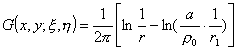3.  椭圆型方程的格林方法

在区域D考虑椭圆型方程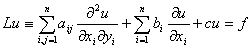[格林函数及其性质]  Lu=0的共轭方程L*u=0的基本解G(x,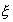)D的边界S上满足

G(x,)=0,  xS1,,n).

格林函数具有对称性质：设G(x,),V(x,)分别为方程Lu=0及其共轭方程的格林函数，则成立对称关系

G(x,)=V(x,)

G(x,)=G(,x)

[利用格林函数解边值问题]

1°  一般公式  在区域D上应用格林公式（本节，四），并取v=G(x,)，则方程Lu=f的狄利克莱问题u|s=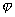的解为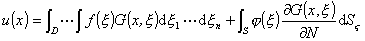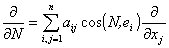NS的外法线方向）

2°  对于球体（球心为O，半径为a），u=0的基本解为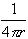rP(x,y,z)与参变点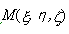的距离，作M关于球面的反演点M1，记r1M1P的距离，则格林函数为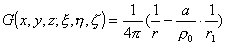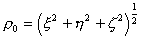.狄利克莱问题u|s=的解为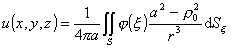3°  在圆上（半径为a），u=0的格林函数为